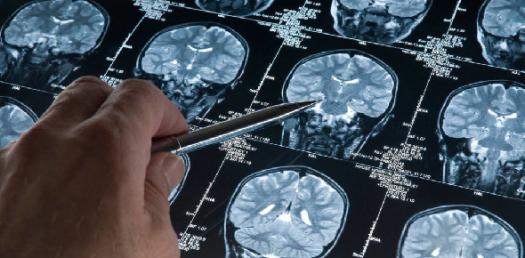# Sacral Diagnosis

44 Questions | Total Attempts: 801Settings.

• 1.
Left Unilateral Flexion: Seated flexion test
• A.

L+

• B.

R+

• C.

Bilateral

• 2.
Left Unilateral Flexion: Sacral sulcus deep
• A.

L

• B.

R

• C.

Bilateral deep

• D.

Bilateral shallow

• 3.
Left Unilateral Flexion: ILA
• A.

L (P/I) or R (A/S)

• B.

R (P/I) or L (A/S)

• C.

Bilateral P/I

• D.

Bilateral A/S

• 4.
Left Unilateral Flexion: Sphinx test
• A.

+

• B.

-

• C.

Equivocal

• 5.
Right Unilateral Flexion: Seated flexion test
• A.

L+

• B.

R+

• C.

Bilateral

• 6.
Right Unilateral Flexion:  Sacral sulcus deep
• A.

L

• B.

R

• C.

Bilateral deep

• D.

Bilateral shallow

• 7.
Right Unilateral Flexion: ILA
• A.

L (P/I) or R (A/S)

• B.

R (P/I) or L (A/S)

• C.

Bilateral P/I

• D.

Bilateral A/S

• 8.
Right Unilateral Flexion:  Sphinx test
• A.

+

• B.

-

• C.

Equivocal

• 9.
Left Unilateral Extension: Seated flexion test
• A.

L+

• B.

R+

• C.

Bilateral

• 10.
Left Unilateral Extension: Sacral sulcus deep
• A.

L

• B.

R

• C.

Bilateral deep

• D.

Bilateral shallow

• 11.
Left Unilateral Extension: ILA
• A.

L (P/I) or R (A/S)

• B.

R (P/I) or L (A/S)

• C.

Bilateral P/I

• D.

Bilateral A/S

• 12.
Left Unilateral Extension: Sphinx test
• A.

+

• B.

-

• C.

Equivocal

• 13.
Right Unilateral Extension: Seated flexion test
• A.

L+

• B.

R+

• C.

Bilateral

• 14.
Right Unilateral Extension: Sacral sulcus deep
• A.

L

• B.

R

• C.

Bilateral deep

• D.

Bilateral shallow

• 15.
Right Unilateral Extension: ILA
• A.

L (P/I) or R (A/S)

• B.

R (P/I) or L (A/S)

• C.

Bilateral P/I

• D.

Bilateral A/S

• 16.
Right Unilateral Extension: Sphinx test
• A.

+

• B.

-

• C.

Equivocal

• 17.
Bilateral Flexion: Seated flexion test
• A.

L+

• B.

R+

• C.

Bilateral

• 18.
Bilateral Flexion: Sacral sulcus deep
• A.

L

• B.

R

• C.

Bilateral deep

• D.

Bilateral shallow

• 19.
Bilateral Flexion: ILA
• A.

L (P/I) or R (A/S)

• B.

R (P/I) or L (A/S)

• C.

Bilateral P/I

• D.

Bilateral A/S

• 20.
Bilateral Flexion: Sphinx test
• A.

+

• B.

-

• C.

Equivocal

• 21.
Bilateral Extension: Seated flexion test
• A.

L+

• B.

R+

• C.

Bilateral

• 22.
Bilateral Extension: Sacral sulcus deep
• A.

L

• B.

R

• C.

Bilateral deep

• D.

Bilateral shallow

• 23.
Bilateral Extension: ILA
• A.

L (P/I) or R (A/S)

• B.

R (P/I) or L (A/S)

• C.

Bilateral P/I

• D.

Bilateral A/S

• 24.
Bilateral Extension: Sphinx test
• A.

+

• B.

-

• C.

Equivocal

• 25.
Left on Right Torsion: Seated flexion test
• A.

L+

• B.

R+

• C.

Bilateral

Related Topics